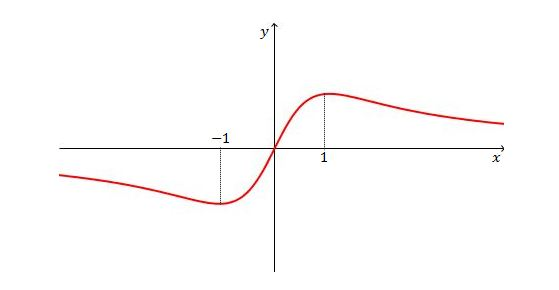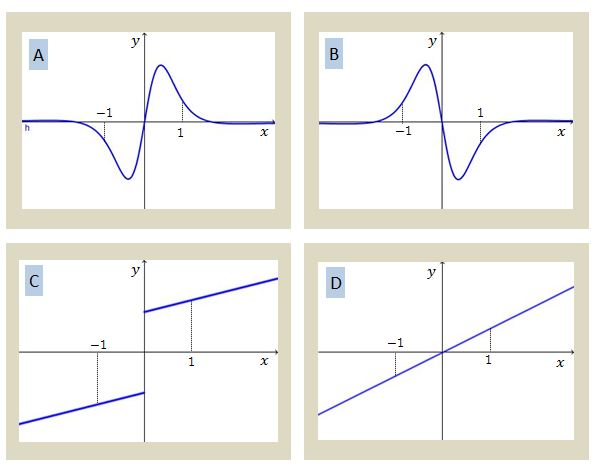# Graphical Second Derivative

Calculus Level 2Given the graph of $y=f(x)$ above, which of the following is a possible graph of $y=f''(x)?$×